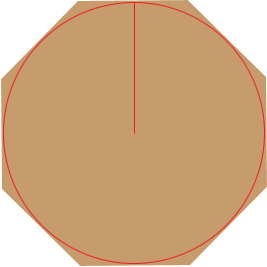SEARCH HOMEMath Central Quandaries & QueriesSubject: Radius of and Inscribed Circle Name: Anna Who are you: Student If I know that the sides of my octagon are 8 units, how do I determine the radius of an inscribed circle?Hi Anna,By the symmetry a line segment from the centre of the circle to the midpoint of a side of the octagon is a radius of the circle. Hence the diameter of the inscribed circle is the width of octagon. If you know the side lengths of a regular octagon then the diameter can be found using the method that I used in the answer to a previous question.

PennyMath Central is supported by the University of Regina and The Pacific Institute for the Mathematical Sciences.